This site uses cookies. By continuing to browse the ConceptDraw site you are agreeing to our Use of Site Cookies.
ConceptDraw Samples | Diagrams — Flowcharts

# Basic Diagramming — Flow Charts

Samples of flowcharts are created with ConceptDraw DIAGRAM v12 diagramming and vector drawing software ConceptDraw DIAGRAM v12 diagramming and vector drawing software enhanced with Basic Diagramming solution from ConceptDraw Solution Park.

ConceptDraw DIAGRAM v12 provides export of vector graphic multipage documents into multiple file formats: vector graphics (SVG, EMF, EPS), bitmap graphics (PNG, JPEG, GIF, BMP, TIFF), web documents (HTML, PDF), PowerPoint presentations (PPT), Adobe Flash (SWF).

## Tutorials and Solutions:

Video Tutorials: ConceptDraw Solution Park

## Sample 1: Flowchart — Import Process

Flow Chart sample: Import process.

This example is created using ConceptDraw DIAGRAM diagramming software enhanced with Basic Diagramming solution from ConceptDraw Solution Park.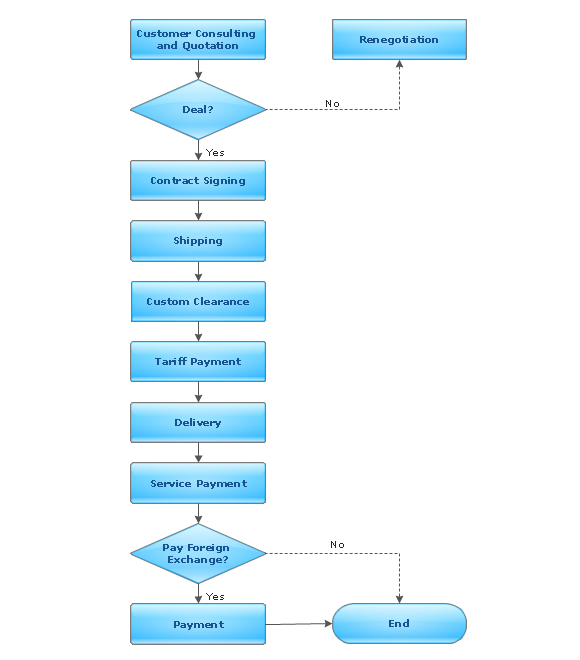## Sample 2: Flowchart — Invoice Payment Process

Flow Chart sample: Invoice payment process.

This example is created using ConceptDraw DIAGRAM diagramming software enhanced with Basic Diagramming solution from ConceptDraw Solution Park.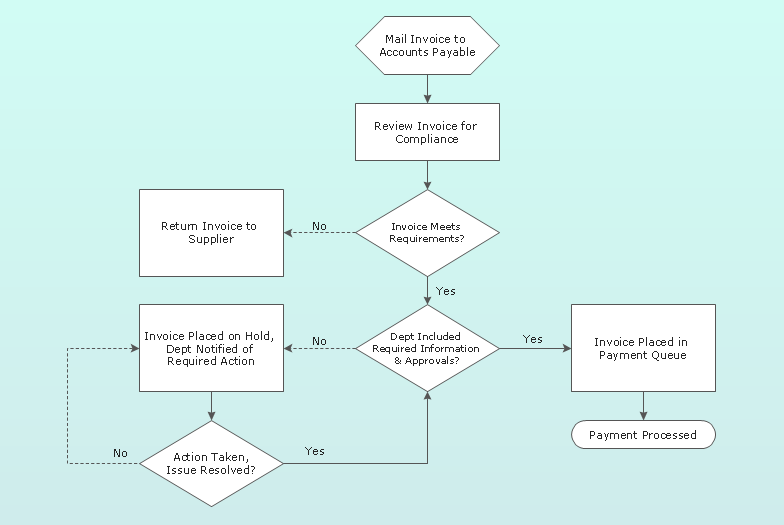## Sample 3: Flowchart Template — Basic Flowchart Symbols

Flowchart template: Basic flowchart symbols.

This example is created using ConceptDraw DIAGRAM diagramming software enhanced with Basic Diagramming solution from ConceptDraw Solution Park.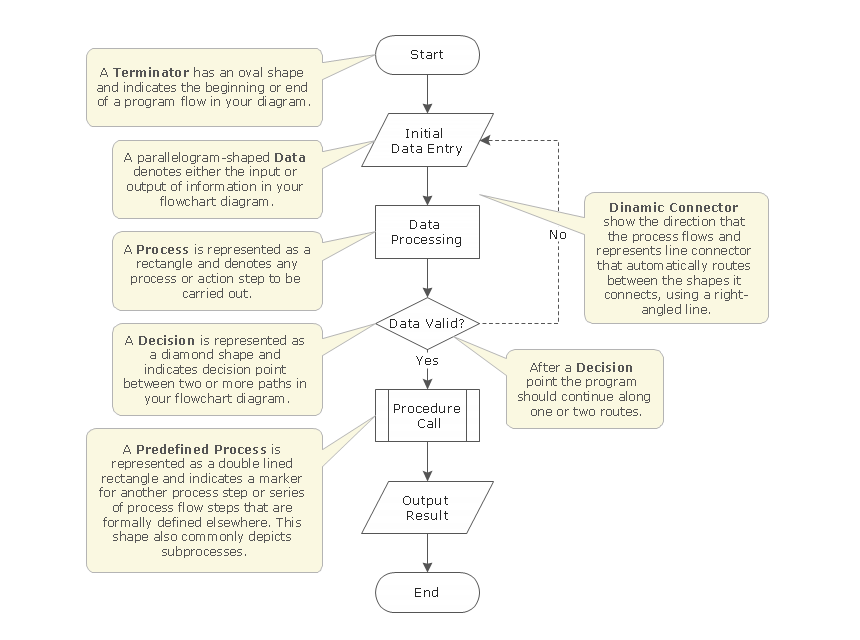## Sample 4: Flowchart — Planning Process

Flow Chart sample: Planning process.

This example is created using ConceptDraw DIAGRAM diagramming software enhanced with Basic Diagramming solution from ConceptDraw Solution Park.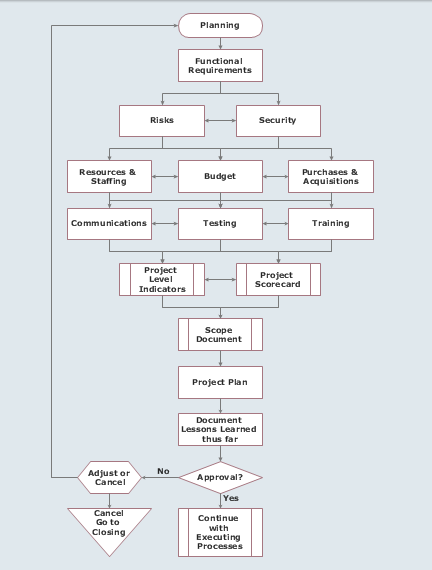## Sample 5: Flowchart — Product Life Cycle Process

Flow Chart sample: Product life cycle process.

This example is created using ConceptDraw DIAGRAM diagramming software enhanced with Basic Diagramming solution from ConceptDraw Solution Park.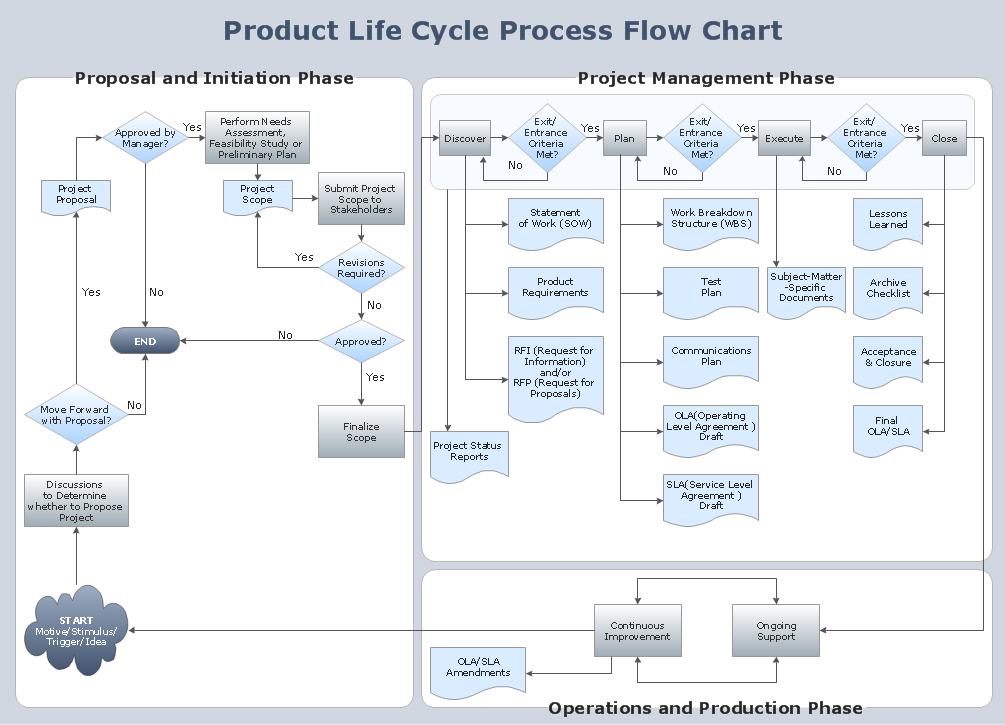## Sample 6: Flowchart — Project Management Life Cycle

Flow Chart sample: Project management life cycle.

This example is created using ConceptDraw DIAGRAM diagramming software enhanced with Basic Diagramming solution from ConceptDraw Solution Park.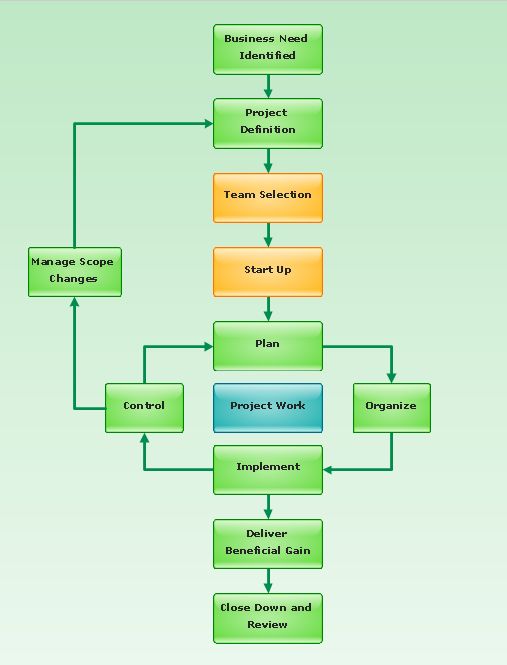## Sample 7: Algorithm Flowchart — Selection Sorting Method

Algorithm Flow Chart sample: Selection sorting method.

This example is created using ConceptDraw DIAGRAM diagramming software enhanced with Basic Diagramming solution from ConceptDraw Solution Park.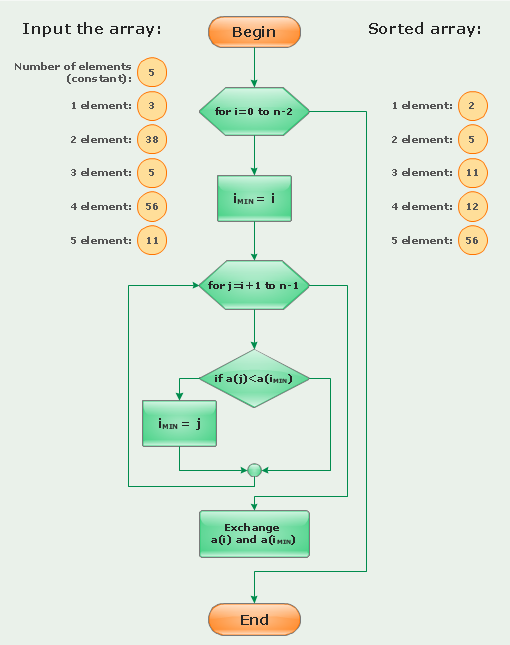## Sample 8: Flowchart — Synthetic Object Construction

Flow Chart sample: Synthetic object construction.

This example is created using ConceptDraw DIAGRAM diagramming software enhanced with Basic Diagramming solution from ConceptDraw Solution Park.## Sample 9: Flowchart — Website Launch

Flow Chart sample: Website launch.

This example is created using ConceptDraw DIAGRAM diagramming software enhanced with Basic Diagramming solution from ConceptDraw Solution Park.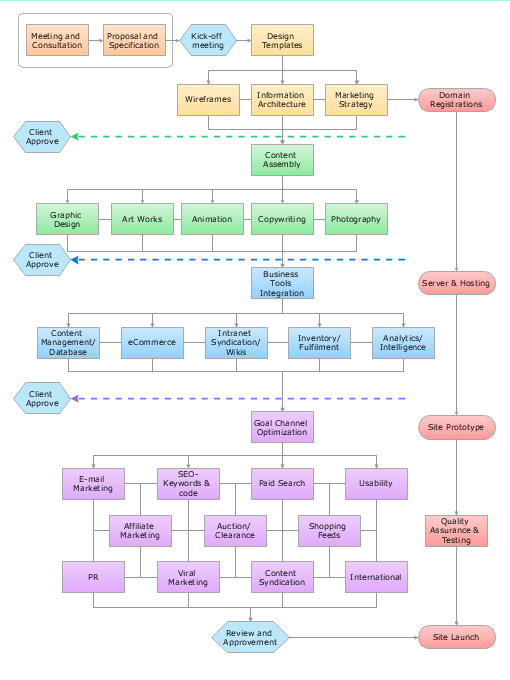## Sample 10: Cross-Functional Flowchart — Credit Approval Process

Cross-Functional Flow Chart sample: Credit approval process.

This example is created using ConceptDraw DIAGRAM diagramming software enhanced with Basic Diagramming solution from ConceptDraw Solution Park.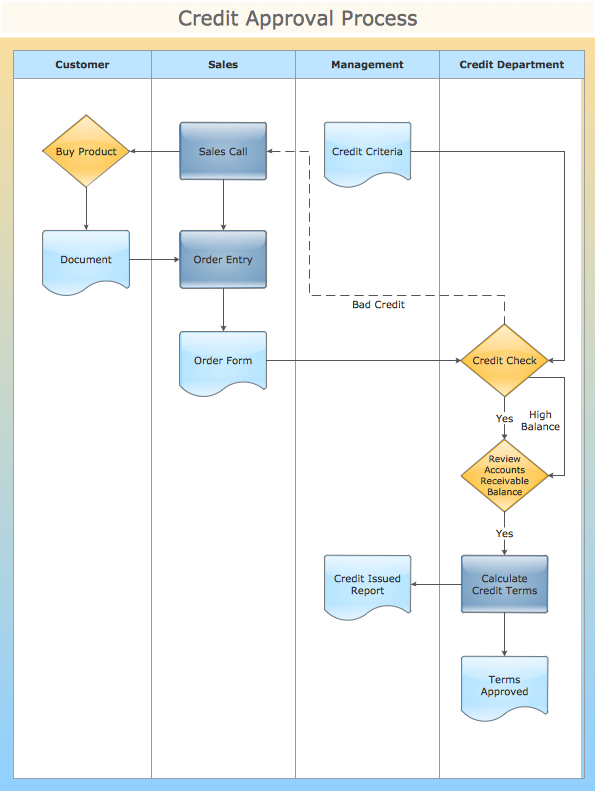## Sample 11: Cross-Functional Flowchart — Ordering Process

Cross-Functional Flow Chart sample: Ordering process.

This example is created using ConceptDraw DIAGRAM diagramming software enhanced with Basic Diagramming solution from ConceptDraw Solution Park.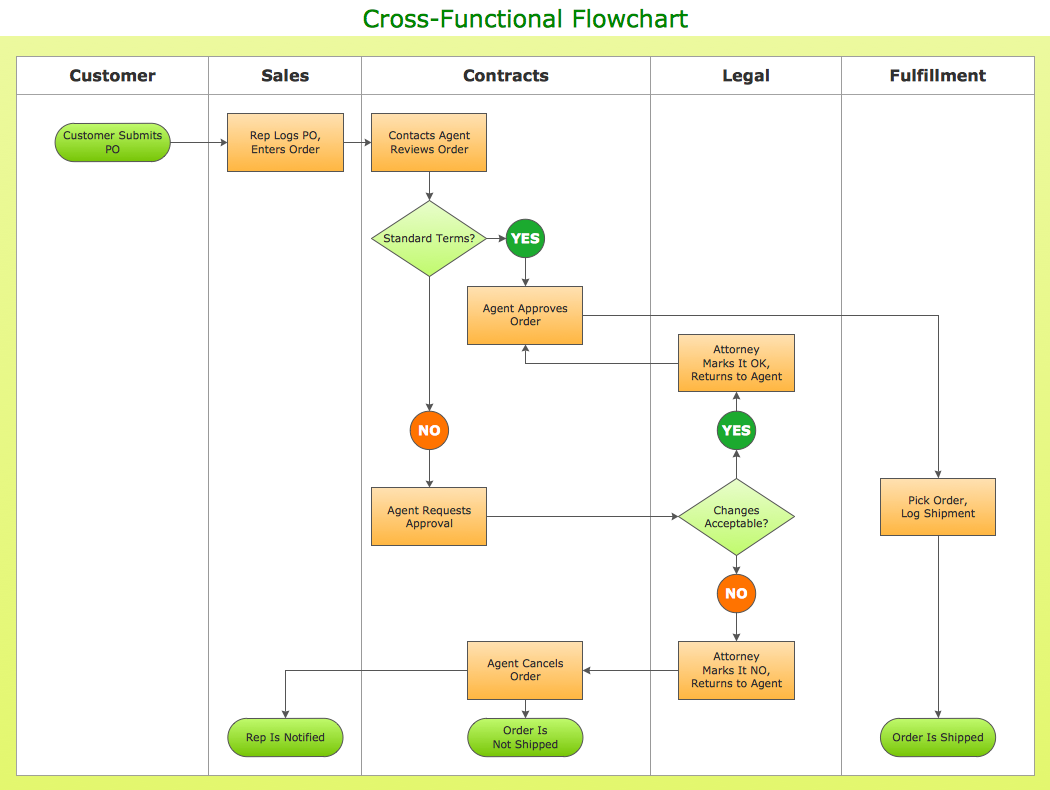## Sample 12: Deployment Flowchart — New Product Development

Deployment Flow Chart sample: New product development.

This example is created using ConceptDraw DIAGRAM diagramming software enhanced with Basic Diagramming solution from ConceptDraw Solution Park.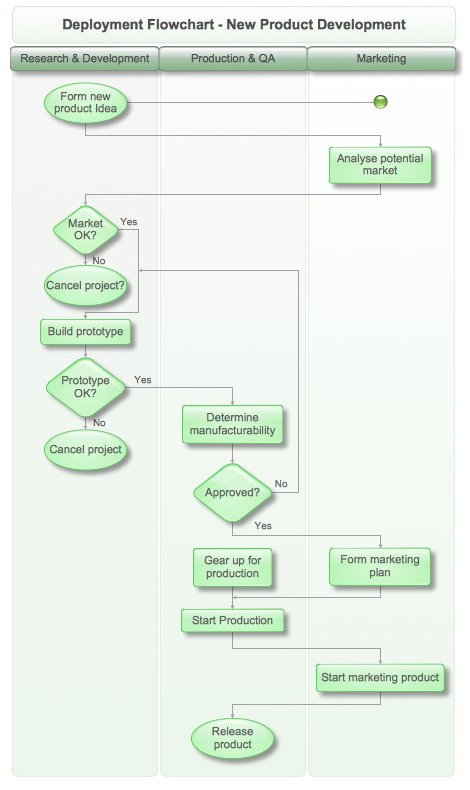## Sample 13: Flowchart — Manufacturing Process

Flow Chart sample: Manufacturing process.

This example is created using ConceptDraw DIAGRAM diagramming software enhanced with Basic Diagramming solution from ConceptDraw Solution Park.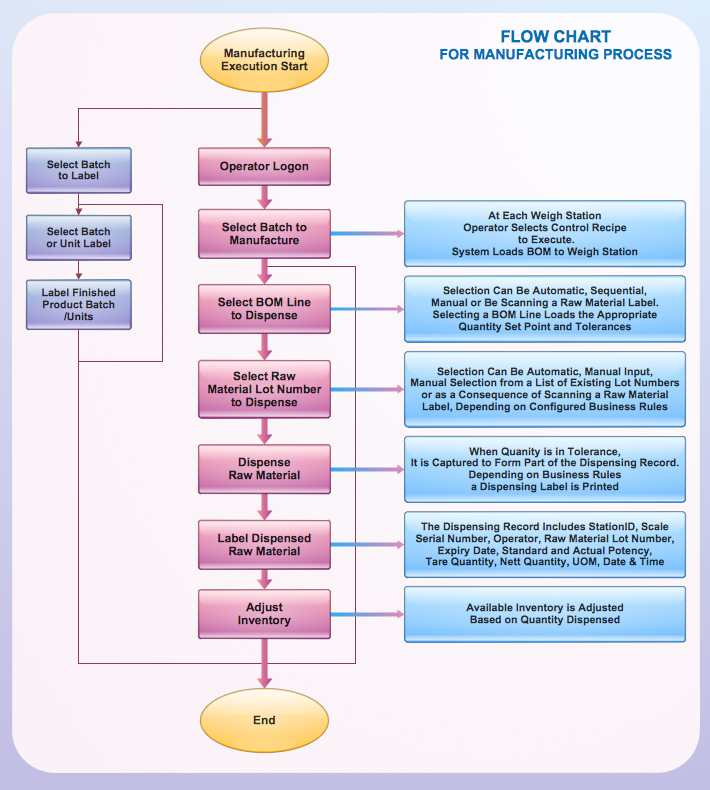## Sample 14: Cross-Functional Flowchart

This example is created using ConceptDraw DIAGRAM diagramming software enhanced with Business Process Diagram solution from ConceptDraw Solution Park.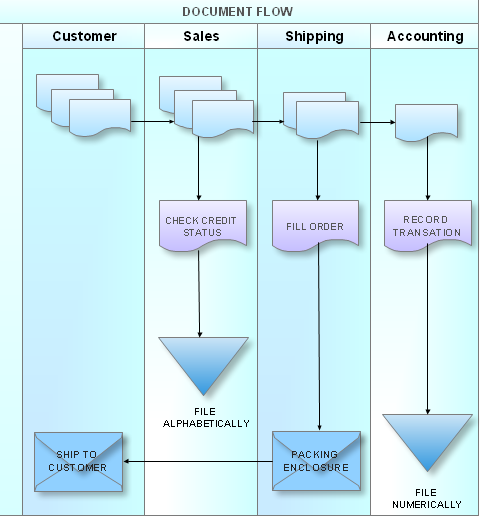All samples are copyrighted CS Odessa's.

Usage of them is covered by Creative Commons “Attribution Non-Commercial No Derivatives” License.

The text you can find at: https://creativecommons.org/licenses/by-nc-nd/3.0